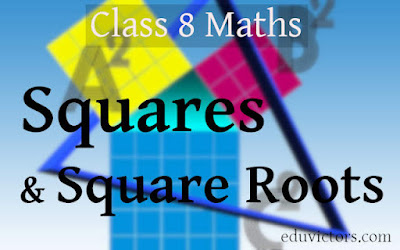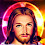# Class 8, NTSE - Maths - Square and Square Roots

### Perfect Square

1. A number is called a perfect square if it is expressed as the square of a number.
2. E.g. 1, 4, 9, 16, 25, ... are called perfect squares (1x1 = 1, 2x2 = 4, 3 x 3 = 9...)
3. In square numbers, the digits at the unit’s place are always 0, 1, 4, 5, 6 or 9.
4. The numbers having 2, 3, 7 or 8 at their units' place are not perfect square numbers.

Q1: Which one of the following numbers is a perfect square:
a) 622
b) 393
c) 5778
d) 625

5. If a number ends with the odd number of zeros then it is not a perfect square.

Q2: Check which of the following is not a perfect square.
a) 81000
b) 8100
c) 900
d) 6250000

Answer: a) 81000 (= 92 x 102 x 10)

6. The square of an even number is an even number while the square of an odd number is an odd number.

7. If n is a positive whole number then (n+1)2 - n2 = 2n + 1
or 2n numbers in between the squares of the numbers n and (n + 1)

Q3: Which of the following perfect square numbers, is the square of an odd number?
289, 400, 900, 1600
a) 289
b) 400
c) 900
d) 1600

Q5: Which of the following perfect square numbers, is the square of an even number?
361, 625, 4096, 65536
a) 361
b) 625
c) 4096
d) 2601

Q6: How many natural numbers lie between squares of 12 and 13.
a) 22
b) 23
c) 24
d) 25

Answer: c) 24 (2x12 = 24)

Q7: A yoga instructor wants to arrange the maximum possible number of 6000 students in the ground so that the number of rows is the same as the number of columns. How many rows will be there if 71 students were left out after the arrangement?
a) 80
b) 88
c) 77
d) 78

Answer: c) 77. (Hint: Remaining students = 6000 - 71 = 5929 = 11 x 11 x 7 x 7 = 11 x 7 = 77)

Q8: The perfect square number between 30 and 40 is:
a)  32
b) 35
c) 36
d) 39

Q9: Can a prime number be a perfect square?
a) True
b) False

8. Unit digits of xn where x, n ∈ W

Units Digit of
Number (x)
Units Digit of
the number (xn)
No. of possibilities
00    1
11    1
22,4,6,8    4
33,9,7,1    4
44,6    2
55    1
66    1
77,9,3,1    4
88,4,2,6    4
99,1    2

Q10: Find the unit digit of (564)64.
a. 2
b. 4
c. 6
d. 8

Hint: Option a and d can be easily eliminated, since 2 and 8 never comes in units place in 4x If you see pattern (4 x 4 = 42 =  16 i.e. 42x = 6 at units place) and (4 x 4 x 4 = 43 = 64 i.e. 42x+1 = 4 at units place). Similarly, (4)64 = 6.

9. The sum of first m odd natural numbers is a perfect square and is equal to m2

10.  12 +  22  +  32  + ...  + n2  = n(n+1)(2n+1)/6

Q11. 1 + 3 + 5 + 7 + 9 + 11 + 13 + 15 is a perfect square of number?
a) 8
b) 7
c) 6
d) 9

Q12: The square of an integer is called a perfect square number. If x is a perfect square number, then its next one is
a. x+1,
b. x2 +1,
c. x2 +2x+1,
d. x+2 √x+1.

Answer: d. (Hint: if x is a perfect square, the number is √x. Next to the perfect square will be (√x + 1)2
i.e. x+2 √x+1.

Q12: As shown in the figure below, the area of three squares are given. Find the perimeter of ΔABC.
a. 12 units
b. 12.5 units
c. 19.5 units
d. 20 units

Answer: a. (Hint: Length of each side of square is √25 = 5, √9 = 3 and √16 = 4. Perimeter = 5+3+4 = 12units)

11. The sum of 1 and the product of any four consecutive integers is a perfect square.
E.g.  3 x 4 x 5 x 6 + 1 =   360 + 1 = 361 = √361 = 19

12. To find the square root of a number, we generally use the following two methods:
• Factorization Method: Finding prime factors of a number
• Long Division method.

Q13: Find the least perfect square number which is divisible 16, 20 and 24?

Answer: Take the LCM of 16, 20 and 24 which is the least number divisible by all three.
i.e. LCM(16,20,24) = 4x4x5x3 = 240
To make 240 perfect square (multiply by 3x5)  = 42 x 52 x 32  = 3600 ...(answer)

______
Q14. Compute √0.0016

Answer: (0.0016) =  (16/10000) = (42 x 10-4 ) = 4 x 10-2 = 0.04

Q15: Prove that product of four consecutive integers plus one is a perfect square number.

Answer: Let the four consecutive integers are: x, x+1, x+ 2 and x+3.
Let P is the product.
⇒ P = x(x+1)(x+2)(x+3)
P = (x2 + x)(x+2)(x+3) = (x3 + 3x2 + 2x)(x+3)  = x4 + 6x3 + 11x2 +  6x
P + 1 = x4 + 6x3 + 11x2 +  6x + 1 = (x2 + 3x + 1)2 ⇒ is a perfect square.

Q16: If  (17)2 is subtracted from a square of a number (x), the result obtained is 1232. Find the number 'x'.

Answer: as per the question, x2 - 172  = 1232
⇒ x2  = 1232 +  172  = 1232 + 289 = 1521
⇒ x = (1521) = 391.How to solve root x - one by root x when x= 9+4root5

1.good question

2.2.good question

3.4.5.Good questions very good

6.ALL QUESTIONS ARE BASED ON THINKING AND APPLICATION SKILL.VERY GOOD